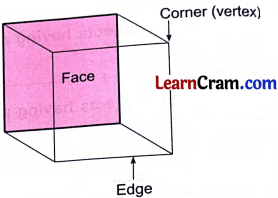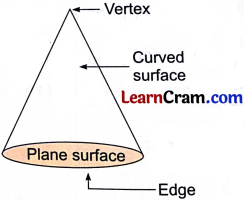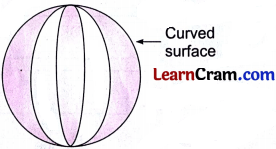# DAV Class 3 Maths Chapter 12 Worksheet 2 Solutions

The DAV Maths Book Class 3 Solutions and DAV Class 3 Maths Chapter 12 Worksheet 2 Solutions of Geometry offer comprehensive answers to textbook questions.

## DAV Class 3 Maths Ch 12 WS 2 Solutions

Fill in the blanks.

Question 1.
A cylinder has ______ edges.
Solution:
Three (2 plane, 1 curved)

Question 2.
A cone has ______ plane surface and ______ curved surface.
Solution:
1, 1

Question 3.
A cuboid has ______ edges and ______ corners.
Solution:
12, 8

Question 4.
The shape of each face of a cube is ______.
Solution:
Square

Question 5.
A cube has ______ faces.
Solution:
6Question 6.
______ faces of a cuboid are identical.
Solution:
Opposite

Question 7.
A sphere has ______ curved surface.
Solution:
1

Question 8.
A cone has ______ edge.
Solution:
1

Question 9.
A cylinder has ______ plane surfaces.
Solution:
2

Question 10.
A sphere has ______ edges and ______ corners.
Solution:
No, No.### DAV Class 3 Maths Chapter 12 Worksheet 2 Notes

Properties of Solid

Cuboid
Cuboid has 6 faces, 12 edges and 8 vertices. All the faces are rectangles and opposite faces look alike.Cube

Cube has 6 faces, 12 edges and 8 vertices. All the faces are squares and all the faces look alike.Cylinder

A cylinder has 2 edges, no comer. It has 2 plane surfaces. It has 1 curved surface.Cone

A cone has 1 edge, 1 vertex. It has 1 plane surface and 1 curved surface.Sphere

A sphere has no edge, no comer. It has 1 curved surface.